### Atoms Class 12th Physics Part Ii CBSE Solution

##### Question 1.Answer the following questions, which help you understand the difference between Thomson’s model and Rutherford’s model better.(a) Is the average angle of deflection of α-particles by a thin gold foil predicted by Thomson’s model much less, about the same, or much greater than that predicted by Rutherford’s model?(b) Is the probability of backward scattering (i.e., scattering of α-particles at angles greater than 90°) predicted by Thomson’s model much less, about the same, or much greater than that predicted by Rutherford’s model?(c) Keeping other factors fixed, it is found experimentally that for small thickness t, the number of α-particles scattered at moderate angles is proportional to t. What clue does this linear dependence on t provide?(d) In which model is it completely wrong to ignore multiple scattering for the calculation of average angle of scattering of α-particles by a thin foil?Answer:(a) The average angle of deflection of α-particles by a thin gold foil predicted by Thomson’s model about the same as that predicted by Rutherford’s model. This is due to the reason that, in both Thomson and Rutherford model of an atom, the average angle of deflection was taken.(b) The probability of backward scattering (i.e., scattering of α-particles at angles greater than 90°) predicted by Thomson’s model much less than that predicted by Rutherford’s model.(c) With increase in the thickness of, the number of target atoms that will increase. This will lead to increase in the collisions, and as we know, scattering is due to single collision. Therefore, with increase in thickness, the chances of collisions increase.Question 2.The gravitational attraction between electron and proton in a hydrogen atom is weaker than the coulomb attraction by a factor of about 10–40. An alternative way of looking at this fact is to estimate the radius of the first Bohr orbit of a hydrogen atom if the electron and proton were bound by gravitational attraction. You will find the answer interesting.Answer:The radius of first Bohr orbit is given by the relation: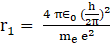……………(1)Where ε0 is the absolute permittivity of free space and is given as = 8.854 × 10-12 N-1C2m-2h is Planck’s constant and is given as 6.64 × 10-34 Jse is charge on an electron and is equals to 1.6 × 10-19Cme is the mass of an electron, and is equals to 9.1 × 10-31 Kgmp is the mass of proton and is equals to 1.67 × 10-27 KgThe forces of coulomb attraction between an electron and proton is given by the relation: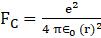Gravitational forces of attraction between an electron and proton is given by the relation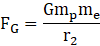Where G is the Gravitational constant and is equal to G = 6.67 × 10-11 N m2 Kg2When coulomb force and Gravitational forces of attraction are equal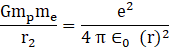Or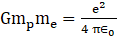…………(1)Substituting the values of equation (1) in equation (2), we get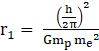Substituting the values, we get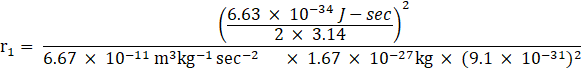On calculating, we get∴ r1= 1.21 × 1029 mSince Universe is 150billion light years wide, which concludes that the radius of first Bohr orbit is much greater than the size of the universe.Question 3.Obtain an expression for the frequency of radiation emitted when a hydrogen atom de-excites from level n to level (n–1). For large n, show that this frequency equals the classical frequency of revolution of the electron in the orbit.Answer:The energy of radiation at level n is given by the relation: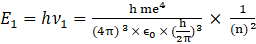…(i)Where v1 is the frequency of radiation at level nH is Planck’s constantm is mass of hydrogen atome is charge on electronε0 is permittivity of free spaceThe energy of radiation at level (n-1) is given by the relation: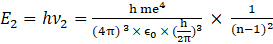……………(ii)Where v2 is the frequency of radiation at level n-1Due to deexcitation of the electrons, the energy released is:E =E2- E1hv = E2- E1…………………..(iii)Putting equation (i) and (ii) in equation (iii), we getv =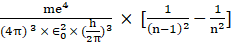v =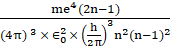For large values of n, (n-1)≈nv =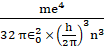Frequency of revolution of an electron is given byVc = v/2πr……………..(vi)Velocity of electron in nth orbit is given by the following relation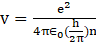…………………….(iv)The radius of nth orbit is given by the following relationr =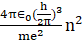………………….(v)Substituting the values of equation (iv) and (v) in equation (vi), we get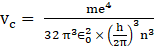Question 4.Classically, an electron can be in any orbit around the nucleus of an atom. Then what determines the typical atomic size? Why is an atom not, say, thousand times bigger than its typical size? The question had greatly puzzled Bohr before he arrived at his famous model of the atom that you have learnt in the text. To simulate what he might well have done before his discovery, let us play as follows with the basic constants of nature and see if we can get a quantity with the dimensions of length that is roughly equal to the known size of an atom (~ 10–10m).(a) Construct a quantity with the dimensions of length from the fundamental constants e, me, and c. Determine its numerical value.(b) You will find that the length obtained in A is many orders of magnitude smaller than the atomic dimensions. Further, it involves c. But energies of atoms are mostly in non-relativistic domain where c is not expected to play any role. This is what may have suggested Bohr to discard c and look for ‘something else’ to get the right atomic size. Now, the Planck’s constant h had already made its appearance elsewhere. Bohr’s great insight lay in recognising that h, me, and e will yield the right atomic size. Construct a quantity with the dimension of length from h, me, and e and confirm that its numerical value has indeed the correct order of magnitude.Answer:(a) Given: charge on an electron and is equals to 1.6 × 10-19Cme is the mass of an electron, and is equals to 9.1 × 10-31 Kgc is speed of light and is equal to 3.0 × 108 m/sThe quantity involving the above quantities is as follows: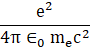1/4πε0 = 9 × 109Nm2C-2Substituting the values we get,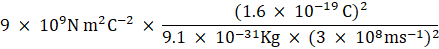On calculating, we get= 2.81 × 10-15mTherefore, the numerical quantity is much smaller than the size of the atom.(b) Given: charge on an electron and is equals to 1.6 × 10-19Cme is the mass of an electron, and is equals to 9.1 × 10-31 KgPlanck’s constant = 6.62 × 10-34JsThe quantity involving the above quantities is as follows:1/4πε0 = 9 × 109Nm2C-2Substituting the values we get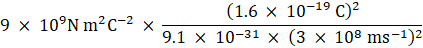On calculating, we get= 2.81 × 10-15mThe numerical quantity is of order of the atomic size.Question 5.The total energy of an electron in the first excited state of the hydrogen atom is about –3.4 eV.(a) What is the kinetic energy of the electron in this state?(b) What is the potential energy of the electron in this state?(c) Which of the answers above would change if the choice of the zero of potential energy is changed?Answer:(a) Given: The total energy of an electron in the first excited state of the hydrogen atom is about –3.4 eV.Kinetic energy of the electron in this state = negative of the total energy = -EKinetic energy of the electron in this state =-(-3.4)eV = + 3.4 eV(b) Potential energy is given as the negative of the twice of the kinetic energyU = -2 (3.4) eVU = -6.8eV(c) If the choice of the zero of potential energy is changed, then the value of potential energy of the system also changes and as we know the total energy is sum of kinetic energy as well as potential energy. Therefore, the potential energy will also changes.Question 6.If Bohr’s quantisation postulate (angular momentum = nh/2π) is a basic law of nature, it should be equally valid for the case of planetary motion also. Why then do we never speak of quantisation of orbits of planets around the sun?Answer:The angular momentum associated with planetary motion is large relative to the Planck’s constant. As the angular momentum of Earth in its orbit is of order of 1070 h which results in higher value of quantum level of order of 1070. The more is the value of n, the less will be the angular momentum and energies. Therefore, the quantum levels of planetary motion are considered as continuous.Question 7.Obtain the first Bohr’s radius and the ground state energy of a muonic hydrogen atom [i.e., an atom in which a negatively charged muon (μ–) of mass about 207me orbits around a proton].Answer:Given: Mass of negatively charged muon mμ– = 207meThe Bohr’s radius is given by the relation:re ∝ (1/mc)Energy of ground state electronic hydrogen atom Ee∝ mThe value of the first Bohr orbit is given as re = 0.53AConverting into metres, re =0.53 × 10-10mLet us consider rμ is the radius of muonic hydrogen atomAt equilibriummμ rμ = me re207me × rμ = me rerμ = (0.53 × 10-10m)/207rμ =2.56 × 10-13mThe ratio of the energies is given as:Ee/Eμ = me/mμ = me/207meWe getEμ = 207EESubstituting the value of energy, we getEμ = 207 × (-13.6)Eμ =-2.81 keV

PDF FILE TO YOUR EMAIL IMMEDIATELY PURCHASE NOTES & PAPER SOLUTION. @ Rs. 50/- each (GST extra)

HINDI ENTIRE PAPER SOLUTION

MARATHI PAPER SOLUTION

SSC MATHS I PAPER SOLUTION

SSC MATHS II PAPER SOLUTION

SSC SCIENCE I PAPER SOLUTION

SSC SCIENCE II PAPER SOLUTION

SSC ENGLISH PAPER SOLUTION

SSC & HSC ENGLISH WRITING SKILL

HSC ACCOUNTS NOTES

HSC OCM NOTES

HSC ECONOMICS NOTES

HSC SECRETARIAL PRACTICE NOTES

# 2019 Board Paper Solution

HSC ENGLISH SET A 2019 21st February, 2019

HSC ENGLISH SET B 2019 21st February, 2019

HSC ENGLISH SET C 2019 21st February, 2019

HSC ENGLISH SET D 2019 21st February, 2019

SECRETARIAL PRACTICE (S.P) 2019 25th February, 2019

HSC XII PHYSICS 2019 25th February, 2019

CHEMISTRY XII HSC SOLUTION 27th, February, 2019

OCM PAPER SOLUTION 2019 27th, February, 2019

HSC MATHS PAPER SOLUTION COMMERCE, 2nd March, 2019

HSC MATHS PAPER SOLUTION SCIENCE 2nd, March, 2019

SSC ENGLISH STD 10 5TH MARCH, 2019.

HSC XII ACCOUNTS 2019 6th March, 2019

HSC XII BIOLOGY 2019 6TH March, 2019

HSC XII ECONOMICS 9Th March 2019

SSC Maths I March 2019 Solution 10th Standard11th, March, 2019

SSC MATHS II MARCH 2019 SOLUTION 10TH STD.13th March, 2019

SSC SCIENCE I MARCH 2019 SOLUTION 10TH STD. 15th March, 2019.

SSC SCIENCE II MARCH 2019 SOLUTION 10TH STD. 18th March, 2019.

SSC SOCIAL SCIENCE I MARCH 2019 SOLUTION20th March, 2019

SSC SOCIAL SCIENCE II MARCH 2019 SOLUTION, 22nd March, 2019

XII CBSE - BOARD - MARCH - 2019 ENGLISH - QP + SOLUTIONS, 2nd March, 2019

# HSCMaharashtraBoardPapers2020

(Std 12th English Medium)

HSC ECONOMICS MARCH 2020

HSC OCM MARCH 2020

HSC ACCOUNTS MARCH 2020

HSC S.P. MARCH 2020

HSC ENGLISH MARCH 2020

HSC HINDI MARCH 2020

HSC MARATHI MARCH 2020

HSC MATHS MARCH 2020

# SSCMaharashtraBoardPapers2020

(Std 10th English Medium)

English MARCH 2020

HindI MARCH 2020

Hindi (Composite) MARCH 2020

Marathi MARCH 2020

Mathematics (Paper 1) MARCH 2020

Mathematics (Paper 2) MARCH 2020

Sanskrit MARCH 2020

Sanskrit (Composite) MARCH 2020

Science (Paper 1) MARCH 2020

Science (Paper 2)

Geography Model Set 1 2020-2021

MUST REMEMBER THINGS on the day of Exam

Are you prepared? for English Grammar in Board Exam.

Paper Presentation In Board Exam

How to Score Good Marks in SSC Board Exams

Tips To Score More Than 90% Marks In 12th Board Exam

How to write English exams?

How to prepare for board exam when less time is left

How to memorise what you learn for board exam

No. 1 Simple Hack, you can try out, in preparing for Board Exam

How to Study for CBSE Class 10 Board Exams Subject Wise Tips?

JEE Main 2020 Registration Process – Exam Pattern & Important Dates

NEET UG 2020 Registration Process Exam Pattern & Important Dates

How can One Prepare for two Competitive Exams at the same time?

8 Proven Tips to Handle Anxiety before Exams!